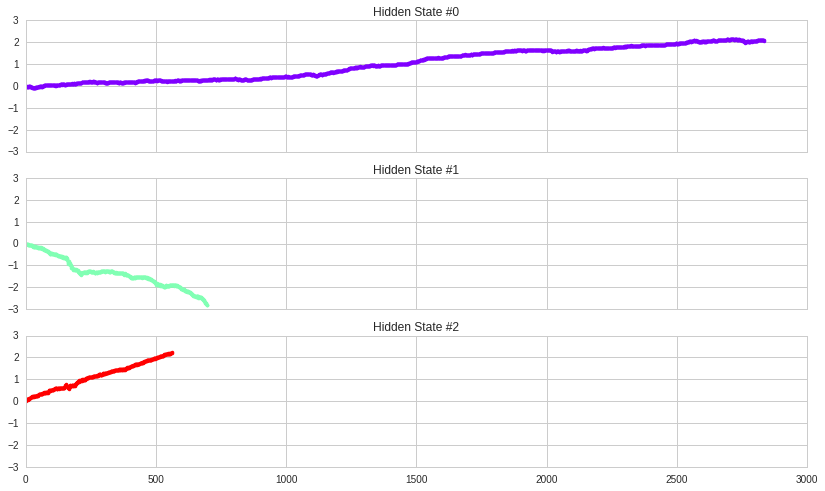Notebook

# Market Regime detetection using PCA/KMeans clustering¶

This is a follow up post on my previous post dealing with market regimes classification in which I had used HMM and SVM on class. This time, I use PCA and KMeans clustering.

For many algorythmic trading strategies, fixed asset allocation weights and model paramters are static and hence, do not adapt to abrupt market regime changes. I believe many algorithms could improve their overall performance by adjusting certain of their paramters based on the current underlying market regime. For example, an algo could bias its weights toward more short positions during market contractions.

The algo uses 1,2,5,10,20,50 day returns and volume as input to classification. Then, it reduces the features using PCA and finally, uses KMeans to classify.

I don't think market regime detection is very useful for predicting upcoming market regimes, however, it can be used in any algo thet needs to have an idea what were the recent past regimes. For example, to adjust length of training sample.

In :
from sklearn import hmm
import pandas as pd
from matplotlib import pyplot as plt
import numpy as np
from quantopian.research.experimental import history
from matplotlib import cm


## PCA and K-Means method¶

In :
df = history(
symbols('SPY'),
fields=['close_price', 'volume'],
frequency='daily',
start='2002-11-19',
end='2019-5-10'
)

In :
df['rets1'] = np.log(df['close_price']) - np.log(df['close_price'].shift(1))
df['rets2'] = np.log(df['close_price']) - np.log(df['close_price'].shift(2))
df['rets5'] = np.log(df['close_price']) - np.log(df['close_price'].shift(5))
df['rets10'] = np.log(df['close_price']) - np.log(df['close_price'].shift(10))
df['rets20'] = np.log(df['close_price']) - np.log(df['close_price'].shift(20))
df['rets50'] = np.log(df['close_price']) - np.log(df['close_price'].shift(50))

df['position'] = np.log(df.reset_index().index.values + 1)
In :
df.dropna(inplace=True)

In :
# X = df[['rets1', 'rets2', 'rets5', 'rets10', 'rets20', 'volume', 'position']].values
X = df[['rets1', 'rets2', 'rets5', 'rets10', 'rets20', 'rets50', 'volume']].values

In :
X.shape

Out:
(4096, 7)
In :
from sklearn.decomposition import PCA
from sklearn.cluster import KMeans

In :
pca = PCA(n_components=3, whiten=True)
X_transformed = pca.fit_transform(X)

In :
pca.components_.shape

Out:
(3, 7)
In :
X_transformed.shape

Out:
(4096, 3)
In :
X = X_transformed

In :
model = KMeans(n_clusters=3)

In :
model.fit(X)

Out:
KMeans(copy_x=True, init='k-means++', max_iter=300, n_clusters=3, n_init=10,
n_jobs=1, precompute_distances='auto', random_state=None, tol=0.0001,
verbose=0)
In :
y = model.predict(X)

In :
plt.scatter(X_transformed[:,0], X_transformed[:,1], c=y)

Out:
<matplotlib.collections.PathCollection at 0x7fce3d705f90>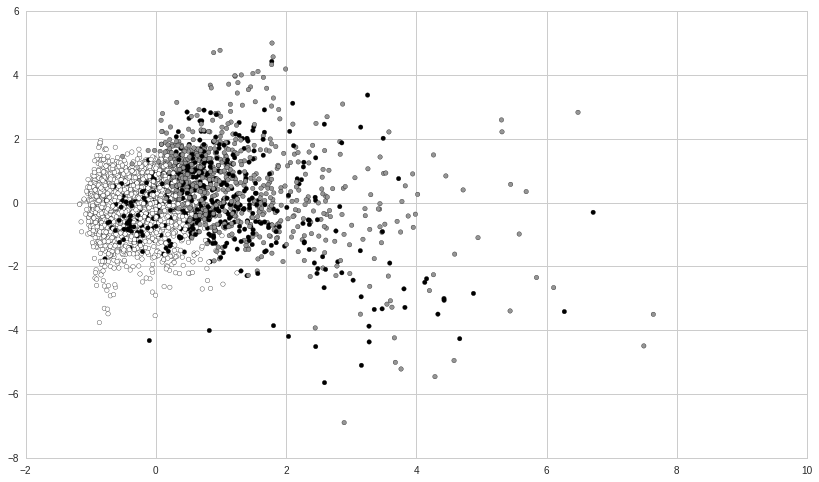In :
def plot_in_sample_kmeans_clusters(model, df):
"""
the in-sample hidden states as a mechanism
to understand the market regimes.
"""
# Predict the hidden states array
hidden_states = model.predict(X)

# Create the correctly formatted plot
fig, axs = plt.subplots(
model.n_clusters,
sharex=True, sharey=True
)
colours = cm.rainbow(
np.linspace(0, 1, model.n_clusters)
)
for i, (ax, colour) in enumerate(zip(axs, colours)):
ax.plot_date(
".", linestyle='none',
c=colour
)
ax.set_title("Hidden State #%s" % i)
ax.grid(True)
plt.show()


### Results¶

It appears that the OCSVM was able to identify market regime changes. Of course, this is all insample.

In :
plot_in_sample_kmeans_clusters(model, df)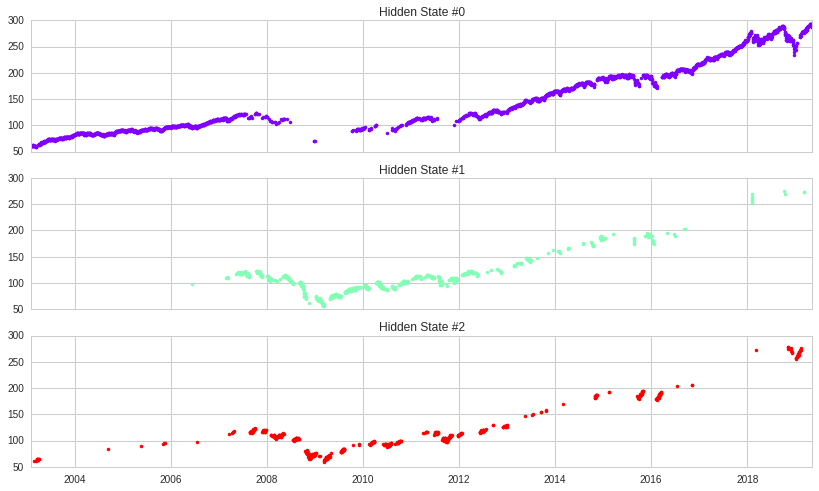In :
y.shape

Out:
(4096,)
In :
df['y'] = y


### Plotting P&L for each regimes¶

In :
print "regime 0 sharpe:", df[df['y'] == 0]['rets1'].mean()/df[df['y'] == 0]['rets1'].std()
print "regime 1 sharpe:", df[df['y'] == 1]['rets1'].mean()/df[df['y'] == 1]['rets1'].std()
print "regime 2 sharpe:", df[df['y'] == 2]['rets1'].mean()/df[df['y'] == 2]['rets1'].std()
print "regime 3 sharpe:", df[df['y'] == 3]['rets1'].mean()/df[df['y'] == 3]['rets1'].std()

regime 0 sharpe: 0.105679855114
regime 1 sharpe: -0.227490580881
regime 2 sharpe: 0.249088474232
regime 3 sharpe: nan


Above, I calculate mean return over risk. Not exactly sharpe. We see that regime 0 is upward trending, 1 is doward trending and 2 & 3 more or less sideways.

In :
# plot of regime 1.
plt.plot(df[df['y'] == 1]['rets1'].cumsum().values)

Out:
[<matplotlib.lines.Line2D at 0x7fce3d448d10>]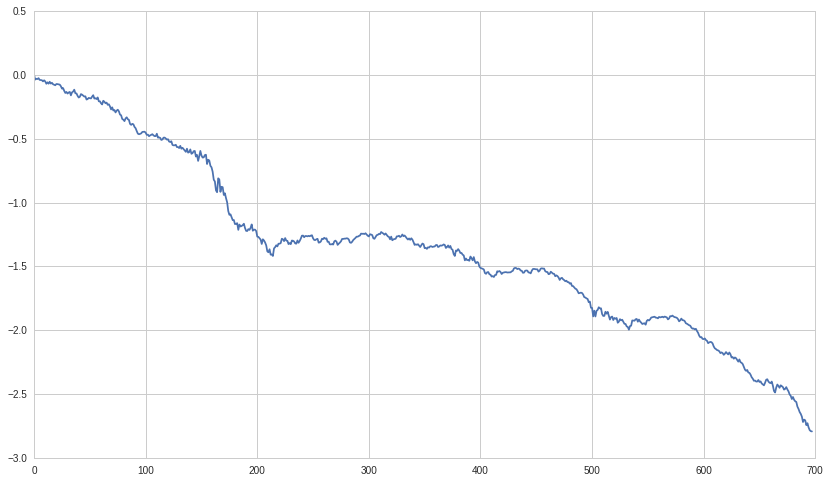In :
# plot of regime 2.
plt.plot(df[df['y'] == 2]['rets1'].cumsum().values)

Out:
[<matplotlib.lines.Line2D at 0x7fce3d19f690>]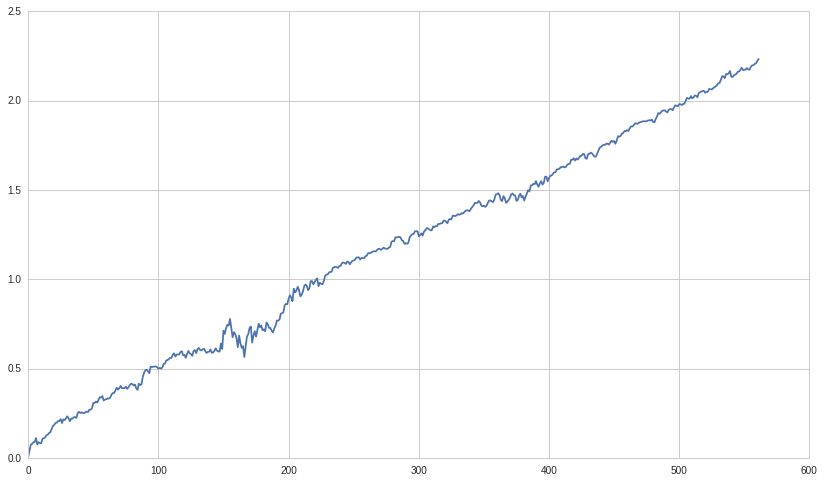In :
fig, axs = plt.subplots(
model.n_clusters,
sharex=True, sharey=True
)
colours = cm.rainbow(
np.linspace(0, 1, model.n_clusters)
)

for i, (ax, colour) in enumerate(zip(axs, colours)):
ax.plot(
df[df['y'] == i]['rets1'].cumsum().tolist(),
".", linestyle='none',
c=colour
)
ax.set_title("Hidden State #%s" % i)
ax.grid(True)
plt.show()